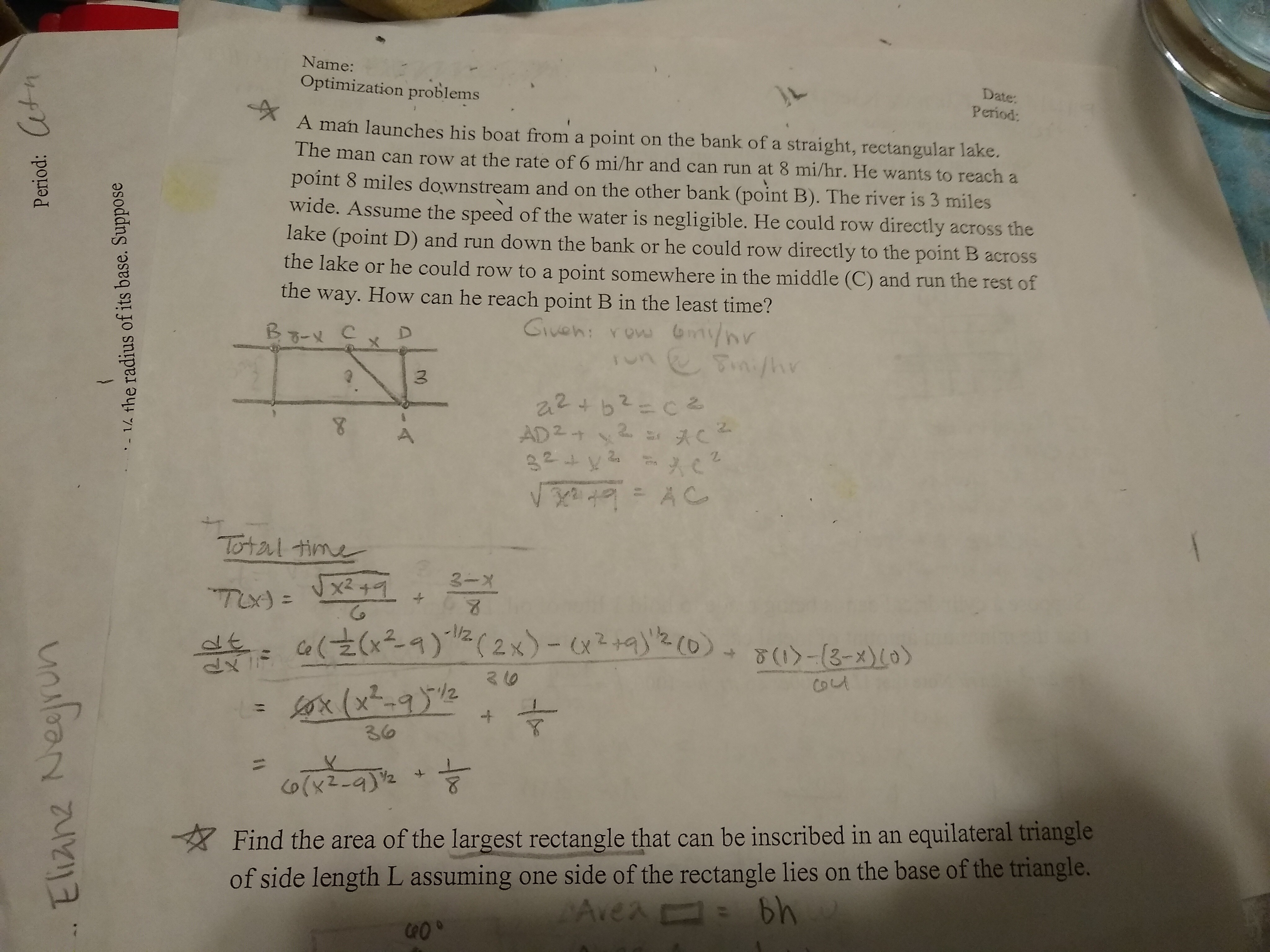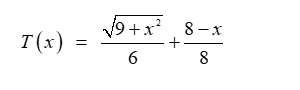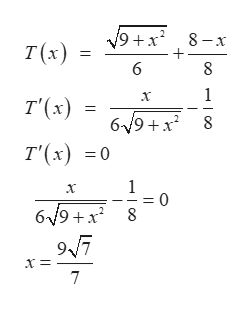# Name:Date:Optimization problemsPeriod:A man launches his boat from a point on the bank of a straight, rectangular lake.The man can row at the rate of 6 mi/hr and can run at 8 mi/hr. He wants to reach apoint 8 miles downstream and on the other bank (point B). The river is 3 mileswide. Assume the speed of the water is negligible. He could row directly across thelake (point D) and run down the bank or he could row directly to the point B acrossthe lake or he could row to a point somewhere in the middle (C) and run the rest ofthe way. How can he reach point B in the least time?Guen: Yow mynrTm/hy2PAD 232C4A ClatexATotal time3-X(2x)- o).( 2x)-292 (0Ca(Cx9)&-13-x)10)3 10(x-92436बहका: हेCo(x2-4)Find the area of the largest rectangle that can be inscribed in an equilateral triangleof side length L assuming one side of the rectangle lies on the base of the triangle.Avea bhathPeriod:unlean aua- 14 the radius of its base. SupposeA

Question
65 views

I'm not sure what do to next at this point. Do I have to find the equation for how long it takes for the man to row from A to C and then to B?help_outlineImage TranscriptioncloseName: Date: Optimization problems Period: A man launches his boat from a point on the bank of a straight, rectangular lake. The man can row at the rate of 6 mi/hr and can run at 8 mi/hr. He wants to reach a point 8 miles downstream and on the other bank (point B). The river is 3 miles wide. Assume the speed of the water is negligible. He could row directly across the lake (point D) and run down the bank or he could row directly to the point B across the lake or he could row to a point somewhere in the middle (C) and run the rest of the way. How can he reach point B in the least time? Guen: Yow mynr Tm/hy 2P AD 2 32 C4 A C latexA Total time 3-X (2x)- o). ( 2x)-292 (0 Ca(Cx9) &-13-x)10) 3 10 (x-92 4 36 बहका: हे Co(x2-4) Find the area of the largest rectangle that can be inscribed in an equilateral triangle of side length L assuming one side of the rectangle lies on the base of the triangle. Avea bh ath Period: unlean aua - 14 the radius of its base. Suppose A fullscreen
check_circle

star
star
star
star
star
1 Rating
Step 1

As in the given question we can see the diagram, with the help of the diagram we find the Objective function: The point C lies in the idle of B and D.

So, the distance between C and D is (CD) = x

Then distance between B and C is (BC) = 8 – (CD) = 8 – x

Now we need to find AC

Here we use Pythagorean theorem:

Step 2

Now the total time as a function of x is:Step 3

Now to maximize the function We find the critical points thr...help_outlineImage Transcriptionclose9+x8-x T(x) 6 8 1 T'(x) 619+x 8 T'(x) 0 1 0 619 +x 8 97 7 fullscreen

### Want to see the full answer?

See Solution

#### Want to see this answer and more?

Solutions are written by subject experts who are available 24/7. Questions are typically answered within 1 hour.*

See Solution
*Response times may vary by subject and question.
Tagged in

### Other